# Universal problems

A concept in category theory. Let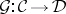be a functor between categories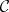and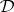, and let. The universal problem defined by this setup requires one to find a "best approximation" ofin, i.e. a universal solution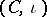consisting of an object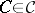and a morphism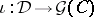insuch that for every object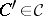and every morphismthere is a unique morphism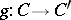such that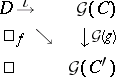commutes.

A universal solution exists if and only if the functor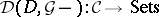is representable (by, cf. Representable functor). There is a universal solution for each choice ofif and only if the functor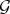has a left adjoint functor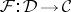. A universal solution of a universal problem is unique up to an isomorphism.

### Examples.

1) Forthe underlying (or forgetful) functor from a category of equationally defined algebras (such as associative algebras, commutative associative algebras, Lie algebras, vector spaces, groups) to the category of sets and for a set, the universal solution is the free algebra over.

2) Forthe functor which associates a Lie algebrawith every associative unitary algebraby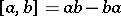and for a Lie algebra, the universal solution is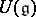, the universal enveloping algebra of.

3) For the imbedding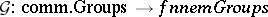and a group, the universal solution is the commutator factor group of(cf. Commutator subgroup).

4) In general, for every underlying (forgetful) functorbetween categories of equationally defined algebras the corresponding universal problems have universal solutions, i.e. there are relatively free objects for any such functor.

5) For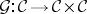the diagonal functor and, the universal problem can be stated in this way: Find an object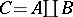inand a pair of morphisms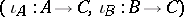in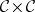such that for any objectand any pair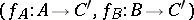there exists a unique morphism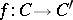such thatcommutes. The universal solution is the coproduct ofand.

6) By considering the dual situation, i.e. by using the categories dual toand, one obtains the dual notions. Forthe diagonal functor and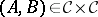, the universal solution of the dual universal problem is the (categorical) product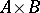ofand.

7) In general, limits and colimits can be obtained as universal solutions of appropriate universal problems.